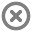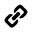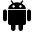Loading...## Info# KS1 Mathematics for Workbook

by Avantis Education UK

176MB

Free## Description

Practical apps, games and tools designed for use in Key Stage One to develop maths skills.

Explore the number system, calculation, fractions, geometry, statistics and more.

This Lesson Profile has been designed with our latest LearnPad device, the Workbook, in mind. It contains no Flash-based resources.

## Contents

• KS1 Number
•Number Pieces
•Number Line
•Number Rack
•Number Frames
•Number Bubble to 100
•Caterpillar Ordering
•Place Value Charts
•Concentration: match different representations of number
•Base Ten BINGO
•Number Grid Fireworks
•Chinese Dragon Number Game
•Okta's Rescue
•Fractions With Trains
•Fraction Matcher
•Guess the Number
•Coconut Ordering
•Paint the Squares
•Chopper Squad
•Helicopter Rescue
•Range Arranger
•Shark Numbers
•Blast Off
•Coconut Odd or Even
•Moving Digits
• KS1 Geometry
•Geoboard
•Pattern Shapes
•Shapes
•Logo
•Symmetry Sorting
•Symmetry Matching
•Colouring tiles
•Shape Patterns
•Tangrams
•SketchBook MobileX
•Symmetry Painter
•Magical Shape Hunt
• KS1 Calculation
•Deep Sea Duel
•Blackboard Times Tables
•Maths Vocabulary Cards
•Number Frames
•Number Line
•Number Pieces
•Hit the Button
•Subtraction Grids
•Number Fact Families
•Maths Lines Addition
•Maths Stack
•Coconut Multiples
•Mental Maths Train
• KS1 Measurement
•StopWatch & Timer
•Time Travel: Learn to tell time
•Order months of the year
•Order days of the week
•Convert Time Units
•Find the Change in Time
•Toy Shop Money
•Coins Game
•Checkout Change Game
•Area Builder
•Coconut Ordering
•Measuring in Centimetres
•Find the Start Time
• KS1 Statistics
•Fuzz Bugs graphing
•Interpret Bar Graphs
•Interpret Tally Charts and Tables
•Which Bar Graph is Correct?
•Create Pictographs
• KS1 Maths Tools
•WorkSpace
•Presenter
•Animator
•Sound Recorder
•Logo
•Camera
•SimpleMind
•Daily 10 Mental Maths Challenge
•Chrome [LP]